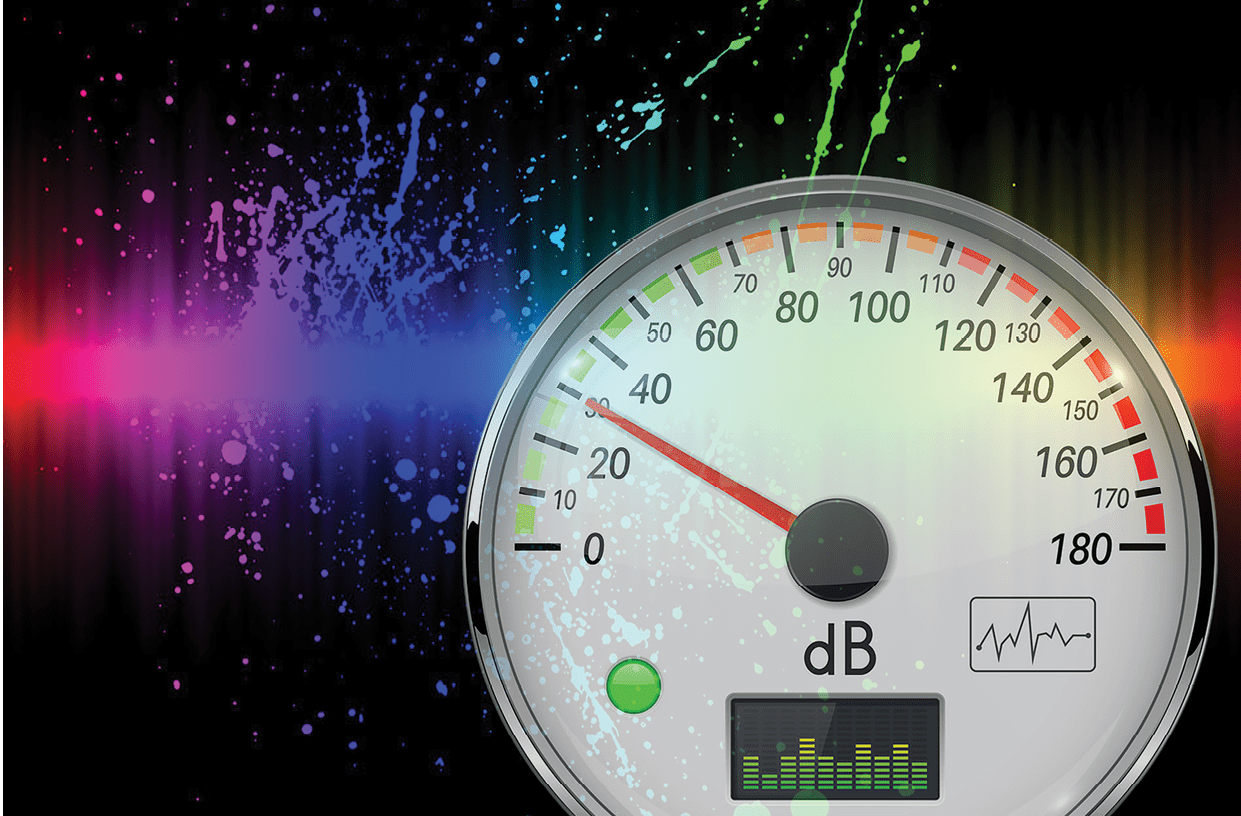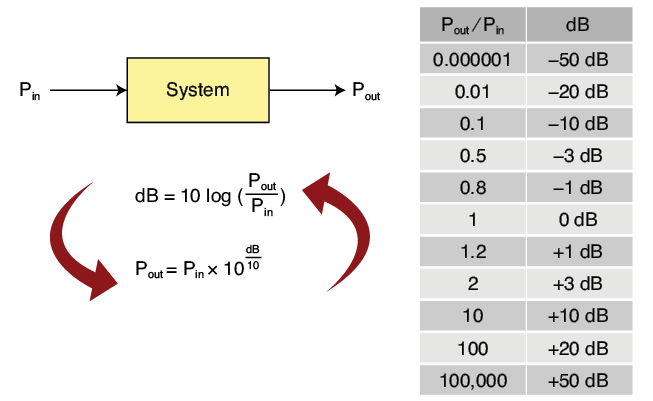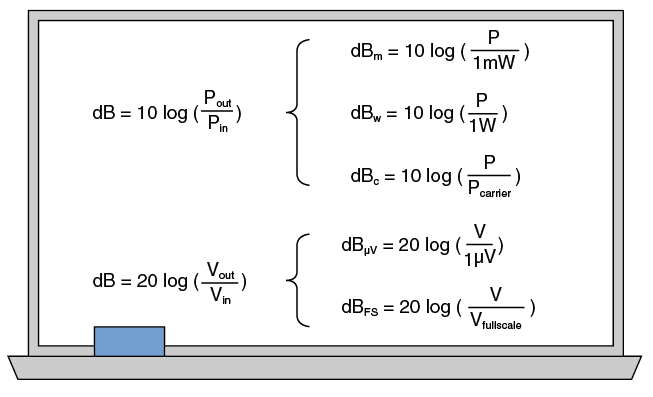# dB for DummiesWritten by

### Decibels Demystified

Understanding decibels—or dB for short—may seem intimidating. Frequent readers of this column know that Robert uses dB terms quite often—particularly when talking about wireless systems or filters. In this article, Robert discusses the math underlying decibels using basic concepts. The article also covers how they are used to express values in electronics, and even includes a quiz to help you hone your decibel expertise.

The decibel is a logarithmic unit, so first I would like to remind you what a logarithm is and what it’s useful for. Once again, breathe normally. Nothing is complex here. For clarity, I’ll only talk about the so-called decimal logarithm, noted “log.”

Ready? Let’s start. Do you remember powers of ten: 100=1, 101=10, 102=100, 103=1,000 and so forth? Remember how it also works for negative powers: 10-1=1/101=0.1, 10-2=0.01 and so on? Now the easy part: the so-called logarithm is simply the reciprocal of this power of 10 function. This means that “log” is the power to which a fixed number—usually 10—must be raised to get a given number:

Therefore log(10)=1 because 101=10, log(100)=2 because 102=100 and so on. Similarly, log(0.01)= -2 because 10-2=0.01 and log(1)=0 because 100=1. Easy, right? The graph of this function, which is defined for any strictly positive number, is shown in Figure 1.Figure 1 – A plot of the decimal logarithm function, which is defined for all strictly positive numbers.

How can this mathematical function be used? For electrical engineers it has two interesting properties. First, a log transforms very large or very small numbers into manageable numbers. For example, do you prefer writing 0.000000002332 or its log, which is simply -8.64? Take your calculator to verify it if you wish.

The other interesting property of the logarithm is that it transforms multiplications into additions. How? You know that 103 × 104= 103+4 =107, right? Although it is a multiplication, the exponents are added. If you think twice, you will understand it means that the log of 103 × 104 is the log of 103 (which is 3) plus the log of 104 (which is 4). Using math notation, this property is written as follows:

Plenty of other properties like this one can be derived easily. For a summary, see the white board I’ve reproduced in Figure 2. I will explain to you in a minute why these properties make life really easy for electrical or RF engineers. Those who are more than 60 years old may recall there were slide rules before electronic pocket calculators—or smartphones nowadays. Basically, a slide rule lets you to multiply and divide by “adding” or “subtracting” lengths of the ruler.

Slide rules are based on log scales and the math properties shown earlier in this article. Figure 3 shows a slide rule from my personal collection and a simple example. Do you want to calculate 3 × 4? Align the “1” of the center sliding scale with the “3“ on the top scale, then look for “4” on the sliding scale. You now simply have to read the corresponding value on the top scale, and you’ll get 12 as expected. Thanks to the slide rule and log scales, you have in fact added log(3) and log(4) and the result is log(3×4)=log(12). Easy, just like I promised!Figure 3 – A slide rule is mainly based on log scales. In this illustration, two log scales are aligned to multiply by 3. For example, the 5 of the bottom scale corresponds to the 15 of the top scale, meaning that 3×5=15.

INTRODUCING dB
Now it’s time to talk about decibels. By definition, a decibel—abbreviated dB—is a unit to measure the ratio of two power levels. More exactly, it is equal to ten times the ratio of two power levels, expressed on a logarithmic scale. A mathematical expression is far easier to understand than the previous sentence:

Figure 4 shows an example where a device generates an output power Pout when a power Pin is applied on its input. Its power gain is therefore Pout/Pin. What is its gain, expressed in dB? Simply:

Dividing both sides of this definition by 10 and applying the inverse log function, which is 10X, allows us to easily find this equivalent expression:

The decibel is one-tenth of a “Bel” (“deci”=1/10), resulting in the “10” in the formula above. A Bel is simply the log of a power ratio—even if the Bel unit is rarely used. According to Wikipedia, this unit was invented in 1924 by Bell Telephone Laboratories, to honor of Alexander Graham Bell (1847-1922), inventor of the first telephone.Figure 4 – A decibel is ten times the log of a power ratio. This table gives useful relationships between power ratios and dBs

So, what does a decibel mean? It is simply a way to express an increase or decrease of power, using a log scale. Remember than log(1)=0? This implies that 0 dB is equivalent to a gain of 1—in other words, no loss or gain of power. +10 dB means that the power gain is 10, +20 dB means that the gain is 100 and so on. Similarly, negative gains in dB mean that there is an attenuation. The output power is lower than the input power. -30 dB means the output power is 1030/10=1,000 times lower than the input. Figure 4 gives some easy-to-remember values in dB. In particular, log(2)=0.301. This implies that a power gain of 2 is roughly +3 dB—easy to remember.

Here is an example of a practical application. Imagine that you have a 100 mW signal, and chain two attenuators valued at -20 dB and -3 dB respectively. What would be the output power? Very simple: -20 dB means /100, and -3 dB means /2, so overall 200. The output power will be 100 mW / 200 = 0.5 mW. Another example? What would be the required gain in dB to go from 0.5 mW back to 100 mW? You can calculate it as:

or conclude that +20 and +3 dB would be needed to compensate -20 and -3 dB.

This short example illustrates a very strong property of decibels: Because the dB is a logarithmic unit, the gain of a chain of stages—when expressed in dB—is simply the sum of the gains of each stage. Therefore, if you chain two amplifiers—each with a power gain of 100—then the overall gain will be 100 × 100, a multiplication. If you use dBs, each stage has a gain of 20 dB, and the overall gain is 20+20 dB, an addition. Of course, this is a direct consequence of the property of logs, which replaces multiplication with addition. Refer to the explanation in Figure 5 if you are a bit lost.Figure 5 – When two stages are chained, their power gains are multiplied. When using decibels, this multiplication became an addition, as shown.

20 RATHER THAN 10?
So, a dB is ten times the log of a power ratio. Fine. However, you may find here and there some equations stating that a dB is twenty times the log of something. Why could it be ten sometimes and twenty on other occasions? The answer is easy, and you shouldn’t be confused. By definition, a dB is always 10 x log(power ratio). However, you may be interested in voltage ratios rather than power ratios (Figure 6). And in that case, the “20” is used. Why? Simply because the relationship between powers and voltages includes a square function. Remember Ohm’s law:
P = V2/R. If you do the math, which is easy, or if you look at Figure 6, you will understand why a gain can also be expressed as:

Two caveats here: First, this equivalence assumes that input and output impedances are the same. If not, the result is different. Second, it is easy to make a mistake and to use 20 rather than 10, or the reverse. For simplicity, I encourage you to always come back to powers, and to always use the actual definition of dB which is with a 10.Figure 6 – Decibels are 10 times the log of a power ratio, and this can also be expressed as 20 times the log of a voltage ratio—at least when source and load resistances are identical.

ABSOLUTE VALUES
A value in dB expresses a gain or loss in power, so it’s a relative value. It is also useful to use a log scale to express absolute quantities—like the actual output power of a radio transmitter. Decibels can be used in that case, but if so, you must define the reference: dB’s as compared to what? For example, you might consider that the reference power is 1 W. If you have a transmitter generating 100 W, then its output power as compared to this reference will be 10 log (100W/1W) = +20 dB. To indicate that the reference is actually 1 W, the unit is then written as “dBW.” The small “w” is a reminder that the reference is 1 W. Similarly, the very common dBm unit indicates, by definition, that we are talking about absolute powers compared to 1 mW:

Very easy, right? So of course, dBm = dBW+30 dB. The same kind of notation exists for plenty of other references, including voltages, sound levels, sound pressure and so on. See Figure 7 for the most common ones that I use.Figure 7 – A small letter after “dB” means that the unit is an absolute measurement, expressed on a log scale. The letter signifies the reference—“m” for 1 mW, “W” for 1 W, “c” for carrier power, and so forth.

WRAPPING UP
I hope you now understand that dB’s are not complicated. Even though they use a strange logarithmic scale, that’s for your benefit—the calculations are far easier! If you want to go a bit further, I encourage you to download and read the application note entitled “dB or not dB” published by A. Winter from Rohde & Schwarz. It makes for a good complement to this introductory article.

And have fun!

As Mahatma Gandhi said, “An ounce of practice is worth a thousand words.” So, after you’ve finished reading this whole article, let’s practice! There are some exercises for you in the Quiz box above. Just for fun and for your own experience, I suggest you to take a piece of paper and a pencil, and try to find the solutions by yourself. You won’t even need a calculator. Then check Answer box.

Q1: What’s 400 mW in dBm?

Q2: What’s 1 mW + 13 dB, in mW?

Q3: What’s 1 mW + 13 dB in dBm?

Q4: What power gain, in dB, is needed to go from 10 µW to 5 W?

Q5: What’s 20 dBm + 20 dBm?

Q6: What’s the voltage gain of an amplifier that has a gain of +46 dB?

Q7: Your oscilloscope has an 8-bit ADC, and you measure an RF signal. What’s the power ratio, in dB, between a full-scale signal and a 1 LSB signal?

Q1: Easy without a calculator: 400 mW=4×100 mW=2×2×10×10 mW. As a power ratio of 10 is 10 dB and a power ratio of 2 is 3 dB, this gives 3+3+10+10=26 dBm. If you prefer to use your calculator, you can also key in 10 × log(400), and the result is 26.

Q2: +13 dB means +10+3 dB, so 1 mW+13 dB=1 mW×10×2=20 mW.

Q3: 1 mW is 0 dBm, so 1 mW+13 dB=0 dBm+13 dB=13 dBm

Q4: With a calculator, just key in 10 log(5/10E-6) and you will get 57 dB. Without a calculator, that’s even easier: From 10 μW to 10 W, this is 6 decades, so +60 dB. 5 W is half of 10 W, so 3 dB less, the result is then 60-3=+57 dB.

Q5: No, it’s not 40 dBm… Here we are adding two powers, not multiplying a power with a gain. So you must convert each power in mW and add them. 20 dBm + 20 dBm = 100 mW+100 mW=200 mW, which is 23 dBm

Q6: Here you can use the equation related to voltage gains, which is dB=20 x log(voltage ratio). So, voltage ratio = 10dB/20=1046/20=102.3=199.53. It’s also easy to do without a calculator, by remembering that voltages are proportional to the square root of power, so their log is divided by 2. Therefore, the voltage ratio is the same as 46/2=23 expressed in dB, and 23=3+10+10, giving a voltage ratio of 2×10×10=200.

Q7: The voltage ratio between a full-scale and a 1 LSB signal on an 8-bit ADC is simply 28. Power is proportional to the square of a voltage, so their power ratio is 22×8=216. Remember that a ratio of 2 is equivalent to 3 dB, so 216 is equivalent to 16×3=48 dB.

For detailed article references and additional resources go to:
www.circuitcellar.com/article-materials

RESOURCES
Rohde & Schwarz | www.rohde-schwarz.com

PUBLISHED IN CIRCUIT CELLAR MAGAZINE • June 2019 #347 – Get a PDF of the issue

 Keep up-to-date with our FREE Weekly Newsletter! Don't miss out on upcoming issues of Circuit Cellar. Subscribe to Circuit Cellar Magazine Note: We’ve made the May 2020 issue of Circuit Cellar available as a free sample issue. In it, you’ll find a rich variety of the kinds of articles and information that exemplify a typical issue of the current magazine. Would you like to write for Circuit Cellar? We are always accepting articles/posts from the technical community. Get in touch with us and let's discuss your ideas.